Question

What is the number of moles of Sodium Chloride (NaCl) that is present in 29.22g sample of it?

1.there are 0.5 moles of NaCl present in a 29.22g sample.

to find moles of a compound you would look at the periodic table to find the mass. in this case, the mass of NaCl is 58.44 g/mol. now, you divide the sample mass of 29.22g by 58.44 g/mol. the grams will cancel each other out and you’re left with 0.5 mol in that sample

hope this helps!

2.minhkhue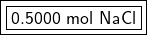Explanation:

We are asked to convert grams to moles. We must use the molar mass or the grams in 1 mole of a substance. These values are found on the Periodic Table as the atomic mass, but the units are grams per mole (g/mol) instead of atomic mass units (amu).

We are given the compound sodium chloride or NaCl. Look up the molar masses of the individual elements.

• Na:  22.9897693 g/mol
• Cl: 35.45 g/mol

The formula for the compound does not contain any subscripts, so there is 1 mole of each element in 1 mole of the compound. Add the molar masses together to find sodium chloride’s molar mass.

• NaCl: 22.9897693 + 35.45= 58.4397693 g/mol

Now we can use the molar mass to create a ratio. We know there are 58.4397693 grams of NaCl in 1 mole of NaCl.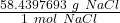Multiply by the number of grams provided in the problem: 29.22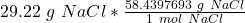Flip the ratio so the units of grams of NaCl cancel.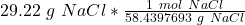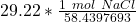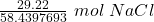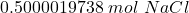The original measurement of grams has 4 significant figures, so our answer must have the same. For our answer, that is the ten-thousandth place. The 0 to the right of this place (0.5000019738) tells us to leave the 0 in the ten-thousandth place.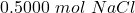There are approximately 0.5000 moles of sodium chloride in a 29.22 gram sample of sodium chloride.